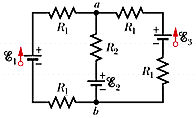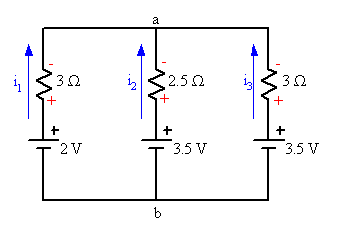# Circuit help - resistors and batteries in a network

circuit help -- resistors and batteries in a network## Homework Statement

(a) Calculate the current through each ideal battery in Fig. 28-41.
(As a sign convention, assume the currents are "up" through each battery.)

A (I1)
A (I2)
A (I3)

Assume that R1 = 1.5 , R2 = 2.5 , ε1 = 2.0 V, and ε2 = ε3 = 3.5 V.

(b) Calculate Va - Vb

V=iR

## The Attempt at a Solution

I dont know how to approach this problem, use kirchhoffs law???

gneill
Mentor

Yes, Kirchhoff's laws or techniques based upon them are the way to go.

ok so i set up 3 loops and after simplifying i get:

Loop 1(the square on the left):-.6+1.2(i1)= i2
Loop 2(right square): 2.5(i2)=1.5(i3)
loop 3(the whole rectangle): 1.5=-3(i1+i2)

and that i1 = i2+i3

gneill
Mentor

ok so i set up 3 loops and after simplifying i get:

Loop 1(the square on the left):-.6+1.2(i1)= i2
Loop 2(right square): 2.5(i2)=1.5(i3)
loop 3(the whole rectangle): 1.5=-3(i1+i2)

and that i1 = i2+i3

Check the signs in your Loop 1 equation.

The coefficient of the i3 term in your Loop 2 equation doesn't look right.

Check the signs of the terms in your Loop 3 equation.

ok i just redid my loops im not sure what is going wrong

1) 2-1.5(i1)-2.5(i2)-3.5-1.5(i1)=0
2) 3.5-2.5(i2)-1.5(i3)-3.5-1.5(i3)=0
3) 2-1.5(i1)-1.5(i3)-3.5-1.5(i3)-1.5(i1)=0

1) -.6-1.2(i1)=i2
2)-.833(i2)=i3
3)-.5-i3=i1

gneill
Mentor

ok i just redid my loops im not sure what is going wrong

1) 2-1.5(i1)-2.5(i2)-3.5-1.5(i1)=0
2) 3.5-2.5(i2)-1.5(i3)-3.5-1.5(i3)=0
3) 2-1.5(i1)-1.5(i3)-3.5-1.5(i3)-1.5(i1)=0

1) -.6-1.2(i1)=i2
2)-.833(i2)=i3
3)-.5-i3=i1

Let's gather together the resistors in the branches and redraw the circuit showing the currents (blue) and the potential drops across the resistors (red) caused by those currents. This should make it easier to keep the signs of things straight when you do your "KVL walk" around a loop.See if you can spot any sign issues in your equations.

#### Attachments

1) -1.5-3(i1)-2.5(i2)=0
2) -2.5(i2)-3(i3)=0
3) -1.5-3(i1)-3(i3)=0

1) 1.5=-3(i1)-2.5(i2)
2)-2.5(i2)=3(i3)
3)1.5=-3(i1)-3(i3)

gneill
Mentor

1) -1.5-3(i1)-2.5(i2)=0
2) -2.5(i2)-3(i3)=0
3) -1.5-3(i1)-3(i3)=0

1) 1.5=-3(i1)-2.5(i2)
2)-2.5(i2)=3(i3)
3)1.5=-3(i1)-3(i3)

Did you pay attention to the directions of the potential changes on the resistors as you passed over them during your "walks" around the loops? I question the sign you've attributed to the 2.5(i2) term in your first loop equation, and to the 3(i3) terms in equations 2 and 3.

Remember, the polarities of the potential changes on the resistors are fixed by the assumed directions of the currents. You record those potential changes according to the direction you pass through the components as you proceed around the loop.